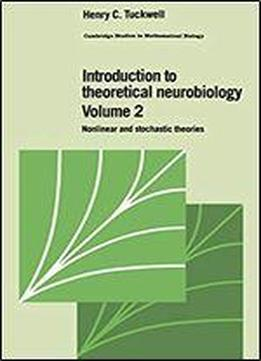# Introduction To Theoretical Neurobiology: Volume 2, Nonlinear And Stochastic Theories (cambridge Studies In Mathematical Biology) by Henry C. Tuckwell / 1988 / English / PDF

The second part of this two-volume set contains advanced aspects of the quantitative theory of the dynamics of neurons. It begins with an introduction to the effects of reversal potentials on response to synaptic input. It then develops the theory of action potential generation based on the seminal Hodgkin-Huxley equations and gives methods for their solution in the space-clamped and nonspaceclamped cases. The remainder of the book discusses stochastic models of neural activity and ends with a statistical analysis of neuronal data with emphasis on spike trains. The mathematics is more complex in this volume than in the first volume and involves numerical methods of solution of partial differential equations and the statistical analysis of point processes.

views: 449